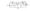# CSVTU Exams Question Papers – Ist Year – Applied Physics-II – 2005

BE (1st Semester)

Examination – 2005

Applied Physics-II

1.(a) What is radioactive decay ? Describe three types of decay fend the law of radioactive decay with mathematical express on. Hence define and derive expression for half-life.

(b) A particle of mass’m’ and charge”‘q’ enters a region of unil Jim magnetic induction field ‘B’ with a velocity. V making an angls 0 with the direction of field. Discuss its motion and defive expressions for:

(i) Radius of path, (ii) Pitch of helical path and (iii) Frequency of Revolution

(c) In a cyclotron the radius of dees is 50 cm and the oscillator frequency is 12 MHz.

(i) Calculate value of magnetic flux density ‘B’ at wlfiich cyclotron attain resonance for proton.

(ii) Calculate the K.E. of emerging proton beam.

(iii) Calculate the value of-’B” for which cyclotron will attain resonace for alpha particle.

(iv) Calculate the maximum velodtyachieved by alpha particles before emerging out of cyclotron dees.

2. (a) What do you understand by coherence? Explain tempor coherence  and spatial coherence.

(b) What is a Hologram ? How does it differ from an ordinary photograph ? Describe in short how a hologram is genera and viewed.

(c) (i) Find the ratio of populations of the two states in a He laser. That (i) produces light of wavelength 620 nm at 27°C. (ii) Numerical aperture of an optical fiber is 0.5 and c refractive index is 1.5. Find refractive index of cladding acceptance angle.

3. (a) What do you understand by Miller indices of a crystal plai Show that the spacing between consecutive parallel plan Defined by Miller indices (h, k, I) is given by:-

Dhki=[h2/a2+k2/b2+l2/c2]1/2

Where a, b and c are primitive vectors along three mut perpendicular axes.

(b) Define energy level and energy band. Explain pi diagrams, how on the basis of Band Theory, solids classified as conductors, insulators and semiconductor

(c) (i) NaCI crystal has FCC structure. The density of Ne 2.18mg/cc. Calculate the distance between two adj atoms. Atomic weight of Na = 23 and At. Wt. of CI = 35.! Calculate the conductivity of pure silicon at temperature when the concentration of carries is 1.6* u crc. CSvenlll«i5oocm2/v x sec and m=5oocm2/v* sec at temperature.

4.(a) What is hall effect ? Derive, expression for hall voltag hall conffecient.

(b) What is superconductivity ? Explain Meissner effect De Type-1 and Type-II superconductors.

(c) In the circuit given below, for CB NPN transistor, ca the value of parameters iEic and vCD(Assume a = 0.99).

FiG:-5. (a) What are polar and Non-polar dielectrics ? Deri\ Mosotti equation for a solid dielectric exhibitin polarizability.

(b) Describe Diamagnetic, paramagnetic and Fe materials. Explain their classification on th< permanent magnetic dipolemoment.

(c) (i) The atomic weight and density of sulphur are gm/c. c. respectively. The electronic polarizat atom is 3.28* lcr”‘ Fm2 .If sulphur crystal symmetry, what will be its relative dielectric consl

(ii) A magnetic material has a magnetization of 23 produces a flux density of 31.4 gauss. Calculate force and relative permeability of the material.